# Heat Exchangers (Part - 5) Notes | Study Heat Transfer - Mechanical Engineering

## Mechanical Engineering: Heat Exchangers (Part - 5) Notes | Study Heat Transfer - Mechanical Engineering

The document Heat Exchangers (Part - 5) Notes | Study Heat Transfer - Mechanical Engineering is a part of the Mechanical Engineering Course Heat Transfer.
All you need of Mechanical Engineering at this link: Mechanical Engineering

8.2.7 Pressure drop in the heat exchanger
Pressure drop calculation is an important task in heat exchanger design. The pressure drops in the tube side as well as shell side are very important and quite a few co-relations are available in the literature. One such co-relation is given below in the subsequent subsection.

8.2.7.1 Correlation for tube side pressure drop (eq. 8.10)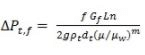(8.10)

where,

ΔPt,f = total pressure drop in the bundle of tube
f  = friction factor (can be found out from Moody’s chart)
Gt = mass velocity of the fluid in the tube
L = tube length
n = no of tube passes
g = gravitational acceleration
ρt = density of the tube fluid
di = inside diameter of the tube
m =0.14 for Re > 2100
0.25 for Re < 2100

The above correlation is for the pressure drop in the tubes owing to the frictional losses. However in case of multi pass flow direction of the flow in the tube changes when flow is from 1-pass to another pass and the pressure losses due to the change in direction is called return-loss. The return-loss (ΔPt,r) is given by eq.8.11,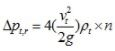(8.11)

n = no of tube pass
vt = velocity of the tube fluid
ρt = density of the tube fluid

Therefore, the total tube side pressure drop will be,

Δpt = ΔPt,f + ΔPt,r

8.2.7.2 Correlation for shell side pressure drop
The following correlation (eq.8.12) may be used for an unbaffled shell,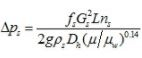(8.12)

The above equation can be modified to the following form (eq.8.13) for a baffled shell,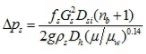(8.13)

where

L = shell length
ns = no of shell pass
nb = no of baffles
ρs   = shell side fluid density
Gs   = shell side mass velocity
Dh  = hydraulic diameter of the shell
Dsi  = inside diameter of shell
fs  = shell side friction factor

The hydraulic diameter (Dh) for the shell can be calculated by the following equation (eq. 8.14),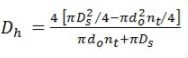(8.14)

where,

nt = number of tubes in the shell
do = outer diameter of the tube
The friction factor (fs) can be obtained by the Moody’s chart for the corresponding Reynolds number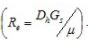8.2.8. Heat transfer effectiveness and number of transfer units (NTU)
The LMTD is required to be calculated for the evaluation of heat exchanger performance. However, the LMTD cannot be directly calculated unless all the four terminal temperatures (Tc,i, Tc,o, Th,i, Th,o) of both the fluids are known.

Sometimes the estimation of the exchanger performance (q) is required to be calculated on the given inlet conditions, and the outlet temperature are not known until q is determined. Thus the problem depends on the iterative calculations. This type of problem may be taken care of using performance equivalent in terms of heating effectiveness parameter (η), which is defined as the ratio of the actual heat transfer to the maximum possible heat transfer. Thus,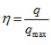(8.15)

For an infinite transfer area the most heat would be transferred in counter-current flow and the qmax will be dependent on the lower heat capacity fluid as such,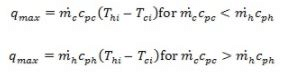The actual heat transfer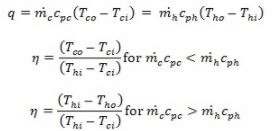The capacity ratio, which is the relative thermal size of the two fluid streams, is defined as,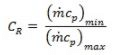On careful analysis, we can say that

U·A: Heat exchange capacities per unit temperature difference.

This thermal sizing (U·A) can be non-dimensionalised by dividing it to the storage capacity of one of the fluid streams. Given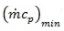limits the maximum heat transfers. The non-dimensional term obtained is known as the number of transfer units (NTU)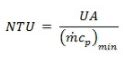It should be noted that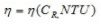The actual determination of this function may be done using heat balances for the streams. For a parallel flow exchanger the relation is shown below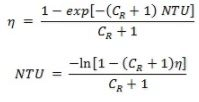The above relation is true for both the condition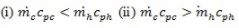Similarly the functional relationship for counter –current exchanger is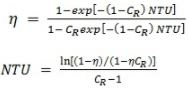(8.16 & 8.17)

The previous relation (eq. 8.16 and 8.17) were for 1-1 exchanger. The relation for 1-2 exchanger (counter current) is given by eq. 8.18, 8.19),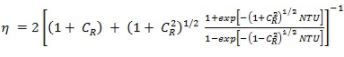(8.18)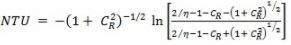(8.19)

When the fluid streams are condensing in a 1-1 pass exchanger (fig. 8.15) as shown below,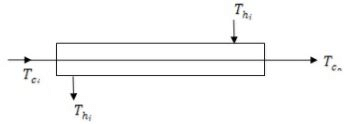Fig.8.15: Condenser with the temperature nomenclature

the following relation arrives.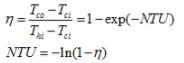The document Heat Exchangers (Part - 5) Notes | Study Heat Transfer - Mechanical Engineering is a part of the Mechanical Engineering Course Heat Transfer.
All you need of Mechanical Engineering at this link: Mechanical EngineeringUse Code STAYHOME200 and get INR 200 additional OFF

## Heat Transfer

58 videos|70 docs|85 tests

Track your progress, build streaks, highlight & save important lessons and more!

,

,

,

,

,

,

,

,

,

,

,

,

,

,

,

,

,

,

,

,

,

;